## Section7.2Inference for paired data

When we have two observations on each person or each case, we can answer questions such as the following:

• Do students do better on reading or writing sections of standardized tests?

• How do the number of days with temperature above 90°F compare between 1948 and 2018?

• Are Amazon textbook prices lower than the college bookstore prices? If so, how much lower, on average?

### Subsection7.2.1Learning objectives

1. Distinguish between paired and unpaired data.

2. Recognize that inference procedures for paired data use the same one-sample $t$-procedures as in the previous section, and that these procedures are applied to the differences of the paired observations.

3. Carry out a complete hypothesis test for paired differences.

4. Carry out a complete confidence interval procedure for paired differences.

### Subsection7.2.2Paired observations and samples

In the previous edition of this textbook, we found that Amazon prices were, on average, lower than those of the UCLA Bookstore for UCLA courses in 2010. It's been several years, and many stores have adapted to the online market, so we wondered, how is the UCLA Bookstore doing today?

We sampled 201 UCLA courses. Of those, 68 required books that could be found on Amazon. A portion of the data set from these courses is shown in Table 7.2.1, where prices are in U.S. dollars.

Each textbook has two corresponding prices in the data set: one for the UCLA Bookstore and one for Amazon. Therefore, each textbook price from the UCLA bookstore has a natural correspondence with a textbook price from Amazon. When two sets of observations have this special correspondence, they are said to be paired.

###### Paired data.

Two sets of observations are paired if each observation in one set has a special correspondence or connection with exactly one observation in the other data set.

To analyze paired data, it is often useful to look at the difference in outcomes of each pair of observations. In the textbook data set, we look at the differences in prices, which is represented as the diff variable. Here, for each book, the differences are taken as

\begin{gather*} \text{ UCLA Bookstore price } - \text{ Amazon price } \end{gather*}

It is important that we always subtract using a consistent order; here Amazon prices are always subtracted from UCLA prices. A histogram of these differences is shown in Figure 7.2.2. Using differences between paired observations is a common and useful way to analyze paired data.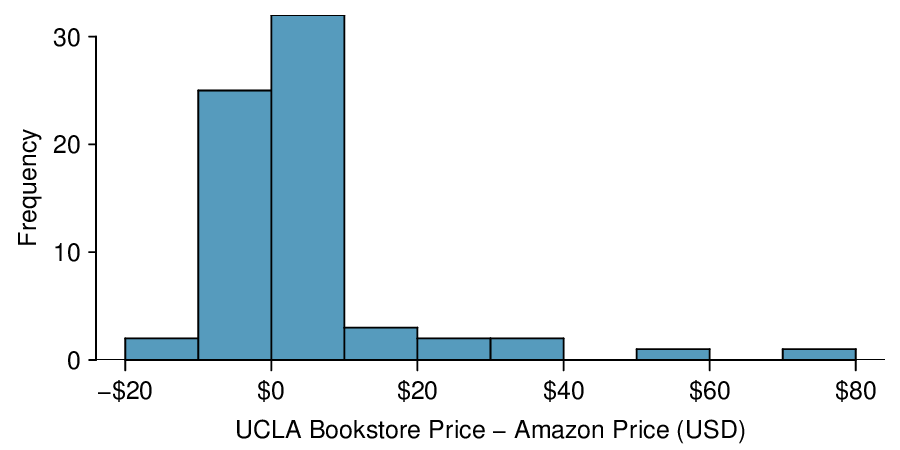Figure 7.2.2. Histogram of the difference in price for each book sampled. These data are very strongly skewed. Explore this data set on Tableau Public.

The first difference shown in Table 7.2.1 is computed as: $47.97 - 47.45 = 0.52\text{.}$ What does this difference tell us about the price for this textbook on Amazon versus the UCLA bookstore?  1

###### Constructing a confidence interval for paired data.

To carry out a complete confidence interval procedure to estimate a mean of differences $\mu_{\text{diff}}\text{,}$

Identify: Identify the parameter and the confidence level, C%.

• The parameter will be a mean of differences, e.g. the true mean of the differences in county population (year 2018 $-$ year 2017).

Choose: Choose the appropriate interval procedure and identify it by name.

• Here we choose the matched pairs $t$-interval.

Check: Check conditions for the sampling distribution of $\bar{x}_{\text{diff}}$ to be nearly normal.

1. There is paired data from a random sample or from a matched pairs experiment.

2. $n_{\text{diff}}\ge 30$ or the population of differences is nearly normal. If the number of differences is less than 30 and the distribution of the population of differences is unknown, check for strong skew or outliers in the sample differences. If neither is found, then the condition that the population of differences is nearly normal is considered reasonable.

Calculate: Calculate the confidence interval and record it in interval form.

• $\text{ point estimate } \ \pm\ t^{\star} \times SE\ \text{ of estimate }\text{,}$ $df\text{:}$ $n_{\text{diff}} - 1$

• point estimate: the sample mean of differences $\bar{x}_{\text{diff}}$

• $SE$ of estimate: $\frac{s_{\text{diff}}}{\sqrt{n_{\text{diff}}}}$

• $t^{\star}\text{:}$ use a $t$-table at row $df = n_{\textit{diff}} - 1$ and confidence level C

• (_______, _______)

Conclude: Interpret the interval and, if applicable, draw a conclusion in context.

• We are C% confident that the true mean of the differences in [...] is between _______ and _______. If applicable, draw a conclusion based on whether the interval is entirely above, is entirely below, or contains the value 0.

An SAT preparation company claims that its students' scores improve by over 100 points on average after their course. A consumer group would like to evaluate this claim, and they collect data on a random sample of 30 students who took the class. Each of these students took the SAT before and after taking the company's course, so we have a difference in scores for each student. We will examine these differences $x_1=57\text{,}$ $x_2=133\text{,}$ ..., $x_{30}=140$ as a sample to evaluate the company's claim. The distribution of the differences, shown in Figure 7.2.13, has a mean of 135.9 and a standard deviation of 82.2. Construct a confidence interval to estimate the true average increase in SAT after taking the company's course. Is there evidence at the 95% confidence level that students score an average of more than 100 points higher after the class? Use the five step framework to organize your work.

Solution

Identify: The parameter we want to estimate is $\mu_{\text{diff}}\text{,}$ the true change in SAT score after taking the company's course. Here, ${\text{diff}}$ = ${\text{ SAT score after course } - \text{ SAT score before course } }\text{.}$ We will estimate this parameter at the 95% confidence level.

Choose: Because we have paired data and the parameter to be estimated is a mean of differences, we will use a matched pairs $t$-interval.

Check: We have a random sample with paired observations and the number of differences is $n_{\text{diff}}=30\ge 30\text{,}$ so we can proceed with the matched pairs $t$-interval.

Calculate: We will calculate the confidence interval as follows.

\begin{gather*} \text{ point estimate } \ \pm\ t^{\star} \times SE\ \text{ of estimate } \end{gather*}

The point estimate is the sample mean of differences: $\bar{x}_{\text{diff}} = 135.9$

The $SE$ of the sample mean of differences is: $\frac{s_{\text{diff}}}{\sqrt{n_{\text{diff}}}} = \frac{82.2}{\sqrt{30}}=15.0$

We find $t^{\star}$ for the one sample case using the $t$-table at row $df = n -1$ and confidence level C%. For a 95% confidence level and $df = 30 - 1 = 29\text{,}$ $t^{\star} = 2.045\text{.}$

The 95% confidence interval is given by:

\begin{align*} 135.9 \ \pm\ \amp 2.045\times \frac{82.2}{\sqrt{30}} \qquad df = 15\\ 135.9 \ \pm\ \amp 2.045\times 15.0\\ =(10\amp 5.2,\ 166.6) \end{align*}

Conclude: We are 95% confident that the true average increase in SAT score following the company's course is between 105.2 points to 166.6 points. There is sufficient evidence that students score greater than 100 points higher, on average, after the company's course because the entire interval is above 100.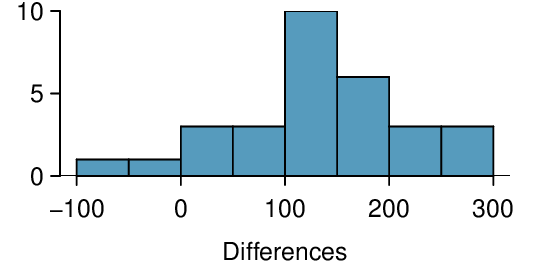Figure 7.2.13. SAT score after course - SAT score before course.

Based on the interval (105.2, 166.6), calculated previously, can we say that 95% of student scores increased between 105.2 and 166.6 points after taking the company's course?  3

No. This interval is attempting to capture the average increase. It is not attempting to capture 95% of the increases. Looking at Figure 7.2.7, we see that only a small percent had increases between 105.2 and 166.6.

### Subsection7.2.6Calculator: the matched pairs $t$-interval

###### TI-83/84: matched pairs T-interval.

Use STAT, TESTS, TInterval.

1. Choose STAT.

2. Right arrow to TESTS.

3. Down arrow and choose 8:TInterval.

4. Choose Data if you have all the data or Stats if you have the mean and standard deviation.

• If you choose Data, let List be L3 or the list in which you entered the differences (don't forget to enter the differences!) and let Freq be 1.

• If you choose Stats, enter the mean, $SD\text{,}$ and sample size of the differences.

5. Let C-Level be the desired confidence level.

6. Choose Calculate and hit ENTER, which returns:

 (,) the confidence interval for the mean of the differences $\bar{x}$ the sample mean of the differences Sx the sample $SD$ of the differences n the number of differences in the sample
###### Casio fx-9750GII: matched pairs T-interval.
1. Compute the paired differences of the observations.

2. Using the computed differences, follow the instructions for a 1-sample $t$-interval.

In our UCLA textbook example, we had 68 paired differences. Because $df=67$ was not on our $t$-table, we rounded the $df$ down to 60. This gave us a 95% confidence interval (0.325, 6.834). Use a calculator to find the more exact 95% confidence interval based on 67 degrees of freedom. How different is it from the one we calculated based on 60 degrees of freedom?  4

Choose TInterval or equivalent. We do not have all the data, so choose Stats on a TI or Var on a Casio. Enter x $= 3.58$ and Sx $= 13.42\text{.}$ Let n $= 68$ and C-Level $= 0.95\text{.}$ This should give the interval (0.332, 6.828). The intervals are equivalent when rounded to two decimal places.
 $n_{\text{diff}}$ $\bar{x}_{\text{diff}}$ $s_{\text{diff}}$ 68 3.58 13.42

### Subsection7.2.7Section summary

• Paired data can come from a random sample or a matched pairs experiment. With paired data, we are often interested in whether the difference is positive, negative, or zero. For example, the difference of paired data from a matched pairs experiment tells us whether one treatment did better, worse, or the same as the other treatment for each subject.

• We use the notation $\bar{x}_{\text{diff}}$ to represent the mean of the sample differences. Likewise, $s_{\text{diff}}$ is the standard deviation of the sample differences, and $n_{\text{diff}}$ is the number of sample differences.

• To carry out inference on paired data, we first find all of the sample differences. Then, we perform a one-sample procedure on the differences. For this reason, the confidence interval and hypothesis test for paired data use the same $t$-procedures as the one-sample methods, where the degrees of freedom is given by $n_{\text{diff}}-1\text{.}$

• When there is paired data and the parameter of interest is a mean of the differences:

• Estimate $\mu_{\text{diff}}$ at the C% confidence level using a matched pairs $t$-interval.

• Test $H_0\text{:}$ $\mu_{\text{diff}}=0$ at the $\alpha$ significance level using a matched pairs $t$-test.

• The conditions for the matched pairs $t$-interval and $t$-test are the same.

1. There is paired data from a random sample or a matched pairs experiment.

2. $n_{\text{diff}}\ge 30$ or the population of differences is nearly normal. If the number of differences is less than 30 and it is not known that the population of differences is nearly normal, we argue that the population of differences could be nearly normal if there is no strong skew or outliers in the sample differences.

• When the conditions are met, we calculate the confidence interval and the test statistic as we did in the previous section. Here, our data is a list of differences.

• Confidence interval:  $\text{ point estimate } \ \pm\ t^{\star} \times SE\ \text{ of estimate }$

• Test statistic: $T = \frac{\text{ point estimate } - \text{ null value } }{SE \text{ of estimate } }$

Here the point estimate is the mean of sample differences: $\bar{x}_{\textit{{diff}}}\text{.}$

The $SE$ of estimate is the $SE$ of a mean of sample differences: $\frac{s_{\textit{{diff}}}}{\sqrt{n_{\textit{{diff}}}}}\text{.}$

The degrees of freedom is given by $df = n_{\textit{{diff}}}-1\text{.}$

### Exercises7.2.8Exercises

###### 1.Air quality.

Air quality measurements were collected in a random sample of 25 country capitals in 2013, and then again in the same cities in 2014. We would like to use these data to compare average air quality between the two years. Should we use a paired or non-paired test? Explain your reasoning.

Solution

Paired, data are recorded in the same cities at two different time points. The temperature in a city at one point is not independent of the temperature in the same city at another time point.

###### 2.True / False: paired.

Determine if the following statements are true or false. If false, explain.

1. In a paired analysis we first take the difference of each pair of observations, and then we do inference on these differences.

2. Two data sets of different sizes cannot be analyzed as paired data.

3. Consider two sets of data that are paired with each other. Each observation in one data set has a natural correspondence with exactly one observation from the other data set.

4. Consider two sets of data that are paired with each other. Each observation in one data set is subtracted from the average of the other data set's observations.

###### 3.Paired or not? Part I.

In each of the following scenarios, determine if the data are paired.

1. Compare pre- (beginning of semester) and post-test (end of semester) scores of students.

2. Assess gender-related salary gap by comparing salaries of randomly sampled men and women.

3. Compare artery thicknesses at the beginning of a study and after 2 years of taking Vitamin E for the same group of patients.

4. Assess effectiveness of a diet regimen by comparing the before and after weights of subjects.

Solution

(a) Since it's the same students at the beginning and the end of the semester, there is a pairing between the datasets, for a given student their beginning and end of semester grades are dependent.

(b) Since the subjects were sampled randomly, each observation in the men's group does not have a special correspondence with exactly one observation in the other (women's) group.

(c) Since it's the same subjects at the beginning and the end of the study, there is a pairing between the datasets, for a subject student their beginning and end of semester artery thickness are dependent.

(d) Since it's the same subjects at the beginning and the end of the study, there is a pairing between the datasets, for a subject student their beginning and end of semester weights are dependent.

###### 4.Paired or not? Part II.

In each of the following scenarios, determine if the data are paired.

1. We would like to know if Intel's stock and Southwest Airlines' stock have similar rates of return. To find out, we take a random sample of 50 days, and record Intel's and Southwest's stock on those same days.

2. We randomly sample 50 items from Target stores and note the price for each. Then we visit Walmart and collect the price for each of those same 50 items.

3. A school board would like to determine whether there is a difference in average SAT scores for students at one high school versus another high school in the district. To check, they take a simple random sample of 100 students from each high school.

###### 5.Global warming, Part I.

Let's consider a limited set of climate data, examining temperature differences in 1948 vs 2018. We randomly sampled 197 locations from the National Oceanic and Atmospheric Administration's (NOAA) historical data, where the data was available for both years of interest. We want to know: were there more days with temperatures exceeding 90°F in 2018 or 1948? 5  The difference in number of days exceeding 90°F (number of days in 2018 - number of days in 1948) was calculated for each of the 197 locations. The average of these differences was 2.9 days with a standard deviation of 17.2 days. We are interested in determining whether these data provide strong evidence that there were more days in 2018 that exceeded 90°F from NOAA's weather stations.

NOAA, www.ncdc.noaa.gov/cdo-web/datasets, April 24, 2019.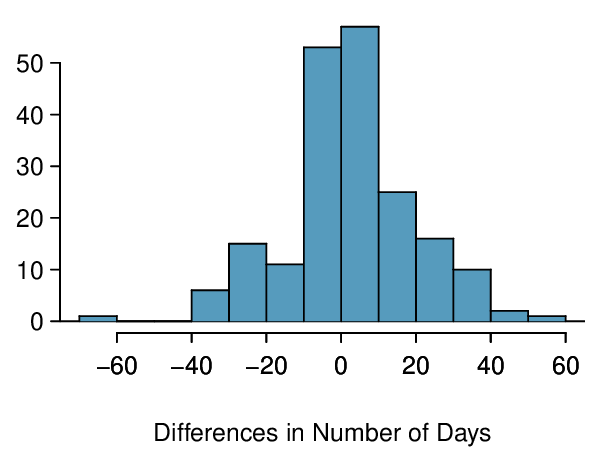1. Is there a relationship between the observations collected in 1948 and 2018? Or are the observations in the two groups independent? Explain.

2. Write hypotheses for this research in symbols and in words.

3. Check the conditions required to complete this test. A histogram of the differences is given to the right.

4. Calculate the test statistic and find the p-value.

5. Use $\alpha = 0.05$ to evaluate the test, and interpret your conclusion in context.

6. What type of error might we have made? Explain in context what the error means.

Solution

(a) For each observation in one data set, there is exactly one specially corresponding observation in the other data set for the same geographic location. The data are paired. Let $diff = 2018 - 1948\text{.}$

(b) $H_{0}: \mu_{diff} = 0$ (On average, the number of days exceeding 90°F in 1948 and 2018 for NOAA stations was the same.) $H_{A}: \mu_{diff} > 0$ (On average, there were more days exceeding 90°F in 2018 than 1948 for NOAA stations.)

(c) Locations were randomly sampled, so independence is reasonable. The sample size is at least 30, so we're just looking for particularly extreme outliers: none are present (the observation off left in the histogram would be considered a clear outlier, but not a particularly extreme one). Therefore, the conditions are satisfied.

(d) $SE = 17.2/ \sqrt{197} = 1.23\text{.}$ $T = \frac{2.9-0}{1.23} = 2.36$ with degrees of freedom $df = 197-1 = 196\text{.}$ This leads to a p-value of about 0.019.

(e) Since the p-value is less than 0.05, we reject $H_{0}\text{.}$ The data provide strong evidence that NOAA stations observed more 90°F days in 2018 than in 1948.

(f) Type 1 Error, since we may have incorrectly rejected $H_{0}\text{.}$ This error would mean that NOAA stations did not actually observe a decrease, but the sample we took just so happened to make it appear that this was the case.

###### 6.High School and Beyond, Part I.

The National Center of Education Statistics conducted a survey of high school seniors, collecting test data on reading, writing, and several other subjects. Here we examine a simple random sample of 200 students from this survey. Side-by-side box plots of reading and writing scores as well as a histogram of the differences in scores are shown below.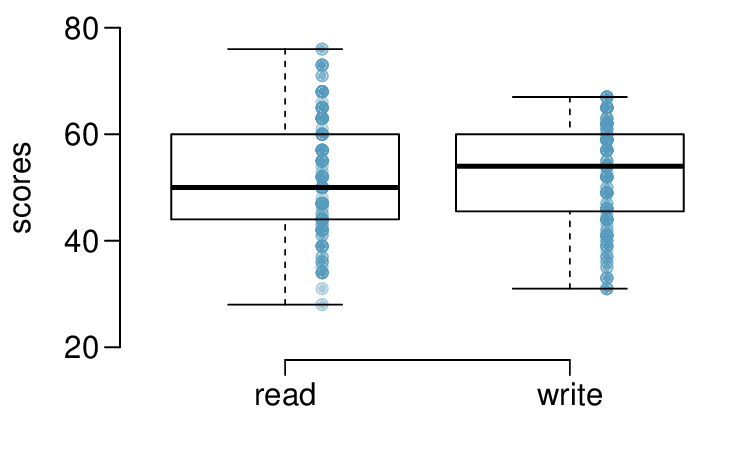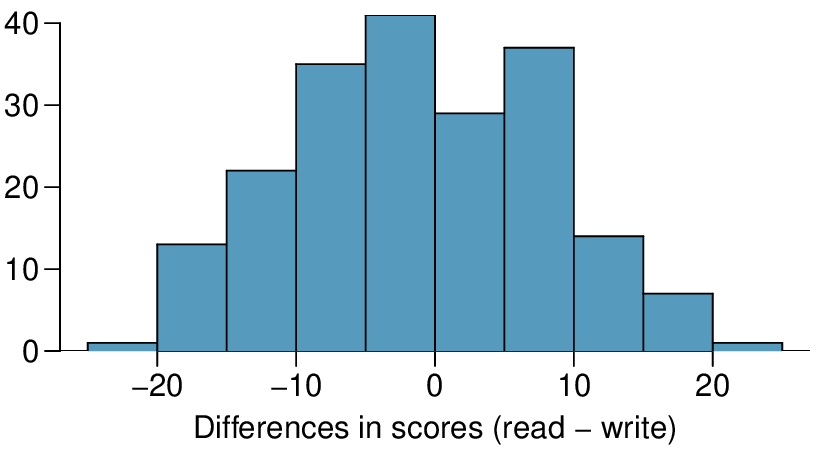1. Is there a clear difference in the average reading and writing scores?

2. Are the reading and writing scores of each student independent of each other?

3. Create hypotheses appropriate for the following research question: is there an evident difference in the average scores of students in the reading and writing exam?

4. Check the conditions required to complete this test.

5. The average observed difference in scores is $\bar{x}_{read-write} = -0.545\text{,}$ and the standard deviation of the differences is 8.887 points. Do these data provide convincing evidence of a difference between the average scores on the two exams?

6. What type of error might we have made? Explain what the error means in the context of the application.

7. Based on the results of this hypothesis test, would you expect a confidence interval for the average difference between the reading and writing scores to include 0? Explain your reasoning.

###### 7.Global warming, Part II.

We considered the change in the number of days exceeding 90°F from 1948 and 2018 at 197 randomly sampled locations from the NOAA database in Exercise 7.2.8.5. The mean and standard deviation of the reported differences are 2.9 days and 17.2 days.

1. Calculate a 90% confidence interval for the average difference between number of days exceeding 90°F between 1948 and 2018. We've already checked the conditions for you.

2. Interpret the interval in context.

3. Does the confidence interval provide convincing evidence that there were more days exceeding 90°F in 2018 than in 1948 at NOAA stations? Explain.

Solution

(a) $SE=1.23$ and $z^{*}=1.65\text{.}$ $2.9 \pm 1.65 \times 1.23 \rightarrow (0.87, 4.93)\text{.}$

(b) We are 90% confident that there was an increase of 0.87 to 4.93 in the average number of days that hit 90°F in 2018 relative to 1948 for NOAA stations.

(c) Yes, since the interval lies entirely above 0.

###### 8.High school and beyond, Part II.

We considered the differences between the reading and writing scores of a random sample of 200 students who took the High School and Beyond Survey in Exercise 7.2.8.6. The mean and standard deviation of the differences are $\bar{x}_{read-write} = -0.545$ and 8.887 points.

1. Calculate a 95% confidence interval for the average difference between the reading and writing scores of all students.

2. Interpret this interval in context.

3. Does the confidence interval provide convincing evidence that there is a real difference in the average scores? Explain.